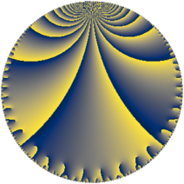# Properties

 Label 76.6.dLevel $76$ Weight $6$ Character orbit 76.d Rep. character $\chi_{76}(75,\cdot)$ Character field $\Q$ Dimension $48$ Newform subspaces $1$ Sturm bound $60$ Trace bound $0$

# Related objects

## Defining parameters

 Level: $$N$$ $$=$$ $$76 = 2^{2} \cdot 19$$ Weight: $$k$$ $$=$$ $$6$$ Character orbit: $$[\chi]$$ $$=$$ 76.d (of order $$2$$ and degree $$1$$) Character conductor: $$\operatorname{cond}(\chi)$$ $$=$$ $$76$$ Character field: $$\Q$$ Newform subspaces: $$1$$ Sturm bound: $$60$$ Trace bound: $$0$$

## Dimensions

The following table gives the dimensions of various subspaces of $$M_{6}(76, [\chi])$$.

Total New Old
Modular forms 52 52 0
Cusp forms 48 48 0
Eisenstein series 4 4 0

## Trace form

 $$48q - 22q^{4} - 4q^{5} - 246q^{6} + 3516q^{9} + O(q^{10})$$ $$48q - 22q^{4} - 4q^{5} - 246q^{6} + 3516q^{9} - 1222q^{16} + 1000q^{17} - 300q^{20} + 17158q^{24} + 24996q^{25} + 12102q^{26} + 6382q^{28} - 16528q^{30} + 27524q^{36} + 3598q^{38} + 35966q^{42} - 65408q^{44} - 13476q^{45} - 143096q^{49} - 148446q^{54} - 53980q^{57} - 117018q^{58} + 133740q^{61} + 28068q^{62} - 78130q^{64} + 128204q^{66} - 48338q^{68} - 104080q^{73} + 48932q^{74} - 251688q^{76} + 184844q^{77} + 80080q^{80} + 375128q^{81} + 175628q^{82} - 384748q^{85} + 139810q^{92} + 183272q^{93} + 601258q^{96} + O(q^{100})$$

## Decomposition of $$S_{6}^{\mathrm{new}}(76, [\chi])$$ into newform subspaces

Label Dim. $$A$$ Field CM Traces $q$-expansion
$$a_2$$ $$a_3$$ $$a_5$$ $$a_7$$
76.6.d.a $$48$$ $$12.189$$ None $$0$$ $$0$$ $$-4$$ $$0$$